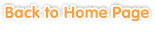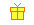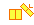Sample questions and games are shown below. Just click to start!Exercises >> Grade 1

Table of Contents
• Chapter 1
From 0 to 10
••
Comparing Numbers and Sequencing•
Addition•
Subtraction•
Addition and Subtraction• Chapter 2
Numbers 11 to 20
•
Counting•
Comparing Numbers and Sequencing••
Addition Up to 20 without Regrouping•
Addition Up to 20 with Regrouping•
Subtraction Up to 20 without Regrouping•
Subtraction Up to 20 with Regrouping•
Addition and Subtraction• Chapter 3
Problem Solving: Numbers up to 20• Chapter 4
Numbers 20 to 100
•
Counting by Groups••
Place Value and Writing Numbers•
Ordinal Numbers•
Adding and Subtracting 10s•
Adding 2-Digit Numbers with 10s•
Adding 2-Digit Numbers with 1-Digit Numbers•
Subtracting 2-Digit Numbers with 10s•
Subtracting 2-Digit Numbers with 1-Digit Numbers•• Chapter 5• Chapter 6• Chapter 7
Patterns
••• Chapter 8
Classifying• Chapter 9• Chapter 10
Telling Time to Hour, Half Hour, and Quarter Hour• Chapter 11
Money
••
Problem Solving• Chapter 12
Reading Graphs and Tables